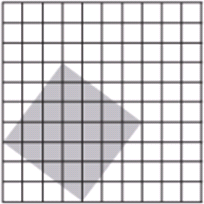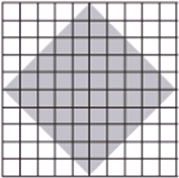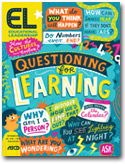Beyond the Right Answer - ASCDSeptember 1, 2015

As students work through challenging mathematical ideas, posing thoughtful questions opens up learning opportunities."I'm confused. How does figuring out the perimeter help us?""We don't know how to count the shaded parts.""We're stuck!"

When math teachers hear students make comments like these, they often wonder how to respond. They might try to craft questions that help students deepen their thinking rather than merely reach the "right" answers. Or they might wonder how to pose questions that encourage students to think like mathematical sense-makers.

Teachers who reply to such comments with questions are on the right track: Posing purposeful questions is an important component of facilitating meaningful discourse in mathematics. Purposeful questions also support another key element of any meaningful math lesson: productive struggle.

## Why Is Struggle Important?

Effective teaching of mathematics provides students both support and opportunities to engage in productive struggle with the content (National Council of Teachers of Mathematics [NCTM], 2014). When students engage in productive struggle, they delve more deeply into understanding relationships among mathematical ideas instead of simply seeking correct solutions. Productive struggle often leads to long-term benefits; with the stronger understanding that arises from such struggle, students can apply their math learning to new problems (Kapur, 2010).

But generating questions that yield productive struggle isn't always intuitive. When replying to comments that clearly signal difficulty ("We're stuck!"), a teacher could well perceive students' frustration or lack of immediate success as indicators that instruction has failed. The teacher might jump in to rescue students by breaking down the task and guiding them step by step (Have you thought about starting by drawing a graph? First, create a table.).

Although well intentioned, this approach deprives students of opportunities to engage fully in making sense of the mathematics (Stein, Smith, Henningsen, &amp; Silver, 2009) or to develop identities as mathematical thinkers capable of reasoning with difficult ideas. A better approach is to pose pointed questions that enable students to engage in struggle that leads to real understanding.

## Questions That Get Students Started

To see such struggle in action, let's drop in on two students, Collin and Marina, as they approach a math problem. The problem (see fig. 1) invites students to look at a shaded figure in relation to a grid and "find the percent, decimal and fractional amount of the shaded area." After solving the task, students discuss their reasoning.

#### Figure 1. Math Task 1: Modeling PercentFind the percentage, decimal, and fractional amount of the shaded area in relation to the whole grid.Source: From Bridges in Mathematics Curriculum (1st ed.) by Math Learning Center. Salem, OR: Author. Copyright Math Learning Center. Used with permission.

As Collin and Marina are working, their teacher, Mr. Craig, comes over to listen:

C<EMPH TYPE="5">ollin: We're stuck.M<EMPH TYPE="5">r. C<EMPH TYPE="5">raig: What do you understand?M<EMPH TYPE="5">arina: That we need to find out how many squares are shaded out of 100 because that will tell us the percent.C<EMPH TYPE="5">ollin: Yeah, but we don't know how to count the little shaded parts.M<EMPH TYPE="5">arina: (tracing her finger around the grey shaded portion) We think the shaded area is a square.M<EMPH TYPE="5">r. C<EMPH TYPE="5">raig: What do you know about finding the area of a square that might help you?

Mr. Craig asks an intentional question (What do you understand about this task?) to help these learners uncover what they already understand about the problem. This question gives them time to make sense of and understand the task and scaffolds their thinking—without the teacher stepping in to do the thinking for them. It also allows Mr. Craig to hear Collin and Marina's current ideas, which helps him to ask a more specific question (What do you know about finding the area of squares?) that scaffolds the struggle. The careful questioning supports Collin and Marina as they think about how to use what they know and encourages them to reflect on and explain their thinking.

To help students engage with a task and support their perseverance in solving problems, pose questions that help students access broad math principles and get a feel for how to approach and think through a rigorous task. Keep questions like these in your "back pocket" to guide students stumped by any math problem:

• What do you already know? What do you need to know?

• What do you understand so far?

• What parts of this problem make sense to you?

• What in this problem doesn't yet make sense to you?

• How might we decide which approach makes more sense?

• What are some math ideas we've worked on before that could help you with this new idea?

## Questions That Make the Mathematics Visible

Teachers can also support productive struggle by asking questions that make the mathematics more visible. For example, let's look at the last question Mr. Craig asked: What do you know about finding the area of a square that might help you? This purposeful question guided Collin and Marina to think specifically about using what they know about finding the area of a square to think about proportion in this problem. In doing so, it built on something they had already noticed (that the shaded area was a square) and sharpened their focus on a mathematically productive strategy without telling them how to solve the problem.

This question also served the mathematical goals for this lesson—to understand that a percentage is a ratio of the whole and that percentages, fractions, and decimals are related because they can all represent the same portion. The question nudged the students toward more sophisticated ways of reasoning—away from counting squares one by one and toward using what they know about decomposing areas of shapes.

These kinds of questions are challenging to pose, in part because they require a deep knowledge of mathematics and an understanding of how students' mathematical ideas build over time. As we have learned through our work with local elementary teachers, a powerful way to generate questions that reveal mathematics concepts is to thoughtfully plan with others who are teaching similar lessons.

When teachers plan collaboratively, they help one another identify the mathematical goal and the types of questions that will make mathematics visible at points of struggle and beyond. They consider how to advance students' mathematical understanding during the lesson and get ready to help students think like mathematical sense-makers (Smith, Bill, &amp; Hughes, 2008).

Questions like these would make the math ideas behind the problem in Figure 1 visible:

• How are percentages, decimals, and fractions related?

• How does that array relate to multiplication and division?

It's important to notice the rich nature of the task these students are working on. Finding the proportion of the area of a shaded figure within a larger figure enables students to engage in active inquiry and exploration.

Tasks that promote reasoning and problem solving are especially fruitful for working on teacher and student questioning during moments of struggle. Such tasks help students engage in meaningful discourse.

Maintaining the high cognitive demand built into these math problems as students grapple with ideas, however, is often challenging (Smith &amp; Stein, 1998). Comments that tell students what to do lower this cognitive demand; students lose opportunities to engage in mathematical reasoning and may pick up unintentional messages that they're not capable of tackling difficult mathematical ideas.

## Encouraging Student-to-Student Questioning

Facilitating meaningful discourse also means supporting student-to-student questioning. Teachers play an essential role in fostering a classroom culture that supports such exchanges. Selecting tasks that warrant discussion, cultivating discussion that centers on making sense of students' ideas, modeling purposeful questions, and orienting students to one another and the mathematics are important steps we can take to give kids opportunities to ask questions of one another and build on ideas collectively.

Consider how Henry and Amaya tackle the same task that Collin and Marina did—figuring out what percentage, decimal, and fraction a shaded portion of a figure represents in relation to a grid—with a larger area shaded (see fig. 2). In presenting this task, Mr. Craig has encouraged students not to count the squares one by one but to use what they know about decomposing areas of regular and irregular polygons to determine the percentage of the grid that's shaded. He hopes they will connect this knowledge to what they know about fractions and decimals.

#### Figure 2. Math Task 2: Modeling Percent.Find the percentage, decimal, and fractional amount of the shaded area in relation to the whole grid.Source: From Bridges in Mathematics Curriculum (1st ed.) by Math Learning Center. Salem, OR: Author. Copyright Math Learning Center. Used with permission.

To help students appreciate that good thinking often emerges from confusion and struggle, he's also suggested to the class that if they get stuck, they can ask one another, "How should we get started?" After Henry and Amaya share brief observations on this tough problem, Henry uses this question to help them move forward.

H<EMPH TYPE="5">enry: I'm thinking it's 25 [small square units]A<EMPH TYPE="5">maya: I'm thinking it's 50.H<EMPH TYPE="5">enry: Let's count it.A<EMPH TYPE="5">maya: Remember, we have to try not to count [the units] one by one.H<EMPH TYPE="5">enry: How should we get started?[Amaya writes "10" on her paper]H<EMPH TYPE="5">enry: So the perimeter is 10? How does figuring out the perimeter help us?A<EMPH TYPE="5">maya: 10 is the number of square units on the edge of the grey part [tracing her finger around the edge of the shaded portion]. I counted 20 half squares, so it's 10 whole, but I don't think 10 is the perimeter—I think it's the total area of the half squares on the edge. What do you think?

Notice how Henry and Amaya found a way to get started on this rigorous task. They both guessed how many small square units were in the shaded portion and then moved into discussing how to calculate what percentage of the whole array the shaded portion takes up. Most important, they persevered in solving the problem. They realized that it's acceptable to not know how to get started, but not acceptable to give up (NCTM, 2014).

## A Vibrant Pursuit

Posing purposeful questions is at the heart of teaching and learning. At the intersection of questioning, productive struggle, and engaging students in meaningful mathematical discourse, we find fascinating territory.

At these points of discontinuity, it's helpful for teachers—and students—to ask open-ended questions (What do you understand so far?) that encourage students to persist and that invite them to develop ideas about how to move forward. It's also fruitful to pose questions that sharpen the focus on the salient mathematics and give learners a way to discuss mathematical structures and make connections among math ideas and relationships (NCTM, 2014). When teachers ask both types of questions, they deepen student thinking and frame mathematics as a more vibrant pursuit than getting a right answer.